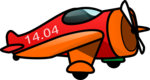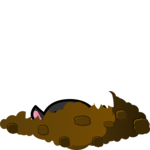# Obeleži sve kategorije koje odgovaraju problemu

### Još detalja - opišite nam problem

Uspešno ste prijavili problem!
Status problema i sve dodatne informacije možete pratiti klikom na link.
Nažalost nismo trenutno u mogućnosti da obradimo vaš zahtev.
Molimo vas da pokušate kasnije.

# Animation by stages¶

## Traffic light¶

One of the best known examples of a device that works by stages is a traffic light. In the example of the traffic light we will explain working by stages and how we can animate events that take place in stages on a computer.

There are several states in which a traffic light can be. For example, it can display red light, flashing yellow light, be turned off, etc. We shall call a period during which the traffic light does not change its state a stage. In normal operation of the traffic light, the stages alternate cyclically and each stage has its own duration. For example, take a traffic light for which the following four stages alternate: 1 - red light, 2 - red and yellow light, 3 - green light, and 4 - yellow light.

To make the animation simpler, we will express the duration of each stage in the number of frames (instead of seconds). Let the durations of the said stages be $$n_1$$, $$n_2$$, $$n_3$$ and $$n_4$$ frames respectively. Then the whole cycle lasts $$N = n_1 + n_2 + n_3 + n_4$$ frames. Of these $$N$$ frames, the first $$n_1$$ belong to the first stage, the next $$n_2$$ to the second stage etc.

To know which stage the current frame belongs to, we can introduce a global variable that will count the frames. Since the whole cycle lasts $$N$$ frames, it is enough to count by modulus $$N$$. This means that when the frame counter reaches the value $$N-1$$, the next value is zero (we only count within one cycle). In this case, for values from 0 to $$n_1 - 1$$, the frame belongs to the first stage, for values from $$n_1$$ to $$n_1 + n_2 - 1$$ to the second stage, for values from $$n_1 + n_2$$ to $$n_1 + n_2 + n_3 - 1$$ to the third stage, and for values from $$n_1 + n_2 + n_3$$ to $$N-1$$ to the fourth stage.

Here’s what a program based on this logic might look like:

####Stage Five: Copy the previous program, then insert a stage for flashing green light, after the green light and before the yellow light (as shown in the example - “Play Task” button).

Hint: In the fifth phase, we will not have a single call to the draw_trafficlights function, but rather a piece of code that looks something like this:

if i_frame % 2 == 0:
draw_trafficlights(...)
else:
draw_trafficlights(...)


####Plane: Write a program that works as shown in the example (“Play Task” button).

Movement description: the plane starts from the center of the left edge of the window. First it moves for 20 frames 2 pixels right and up, then 20 frames 2 pixels right and down. When it comes out through the right edge of the window, it appears at the same height on the left edge. Frame rate is 50 frames per second.####Mole: Write a program that works as shown in the example (“Play Task” button).

10 images are loaded with the mole coming out of the hole a bit more in each image. The cycle has four stages lasting 28 frames in total.

• Stage one lasts 10 frames and during this stage the mole is coming out of the hole (the images are shown in order, from first to tenth).

• Stage two lasts 5 frames during which the mole is in the highest position (the tenth image is shown).

• Stage three lasts 10 frames and during this stage the mole is coming into the hole (the images are shown from tenth to first).

• Stage four lasts 3 frames and during it the mole is in the hole (the first image is shown).